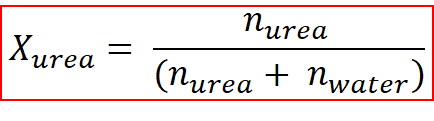# Problem: Find the mass of urea (CH4N2O) needed to prepare 50.1 g of a solution in water in which the mole fraction of urea is 7.66×10−2.

###### FREE Expert Solution

Mole fraction is the ratio of the number of moles of one substance over the total number of moles that are presentThis can be presented as below where the mole ratio of urea in the solution can be found.where,

Xurea is the mole ratio of urea present in the solution and nurea and nwater are the number of moles of urea and water that is present in the solution.

As we know, the mole fraction of urea in the solution to be made is to equal to,

χurea = 7.66 x 10-2  0.0766

96% (216 ratings)###### Problem Details

Find the mass of urea (CH4N2O) needed to prepare 50.1 g of a solution in water in which the mole fraction of urea is 7.66×10−2.